Peliński, K., and Smardzewski, J. (2019). "Experimental testing of elastic properties of paper and WoodEpox® in honeycomb panels," BioRes. 14(2), 2977-2994.

#### Abstract

The literature lacks comparisons of analytical and numerical calculations that have been verified experimentally for elastic constants of auxetic cells in cores manufactured from wood materials. The aim of this study was to determine the effect of auxetic cell geometry and the type of material used in their manufacture on elastic properties of the honeycomb panel core. This paper describes properties of the materials, from which core cells were modeled and presents mathematical models of cell properties. The method of numerical optimization of cell shape was specified, and the numerical calculations concerning modeled cells are given along with the course and results of experimental tests. Additionally, the results of analytical, numerical, and experimental tests were compared. Cell geometry had a considerable effect on elastic properties of honeycomb panel cores, particularly the angle of the cell wall. Moreover, geometric imperfections had a significant effect on the results of analytical calculations. Based on numerical calculations, satisfactory consistency between these results and experimental tests was obtained.

Experimental Testing of Elastic Properties of Paper and WoodEpox® in Honeycomb Panels

Krzysztof Peliński* and Jerzy Smardzewski

The literature lacks comparisons of analytical and numerical calculations that have been verified experimentally for elastic constants of auxetic cells in cores manufactured from wood materials. The aim of this study was to determine the effect of auxetic cell geometry and the type of material used in their manufacture on elastic properties of the honeycomb panel core. This paper describes properties of the materials, from which core cells were modeled and presents mathematical models of cell properties. The method of numerical optimization of cell shape was specified, and the numerical calculations concerning modeled cells are given along with the course and results of experimental tests. Additionally, the results of analytical, numerical, and experimental tests were compared. Cell geometry had a considerable effect on elastic properties of honeycomb panel cores, particularly the angle of the cell wall. Moreover, geometric imperfections had a significant effect on the results of analytical calculations. Based on numerical calculations, satisfactory consistency between these results and experimental tests was obtained.

Keywords: Auxetic; Periodic core structures; WoodEpox®; Experiment; FEM

Contact information: Department of Furniture Design, Faculty of Wood Technology, Poznan University of Life Sciences, Poznan, Poland; *Corresponding author: pelinski@up.poznan.pl

INTRODUCTION

This study determined the effect of the geometry of auxetic cells and the type of material used in their manufacture on elastic properties of cores for honeycomb panels. Moreover, the analytical and numerical calculations for Young’s moduli and Poisson’s ratios were compared with results of experimental tests. First, the properties of materials used to model the core cells were described. The mathematical models describing cell properties and the numerical optimisation of cell shape were presented. After determining the numerical computations for the modeled cells, the results of analytical, numerical and experimental analyses were compared.

EXPERIMENTAL

Materials

Tests were conducted using Testliner 2 paper of 0.15 mm in thickness and grammage of 123 g/m2 manufactured by HM Technology (Brzozowo, Poland). Moreover, WoodEpox® Abatron wood-based composite, density: 430 kg/m3 (Abatron, Kenosha, USA) was also used. According to the manufacturer it is a wood-based material; its composition is entirely based on natural resins (zero VOC emissions and Greenguard® certificate). Elastic properties of paper, including Young’s moduli MOEx and MOEy, moduli of rupture MORx (MPa) and MORy (MPa) as well as Poisson’s ratios, ϑyx and ϑxy, for the machine direction (Y) and the cross-machine direction (X) were determined following the requirements of PN-EN ISO 1924-2 (2010). Tests were conducted on 10 strips of paper of 15 mm in width, from the original length of 180 mm. In order to determine Young’s modulus, MOEy (MPa) and MORy (MPa) in WoodEpox®, tests were conducted on 10 dumbbell-type samples of 5.0 mm ± 0.1 mm in thickness, gauge length of 24 mm ± 0.1 mm, and total length of 235 mm. The testing procedures followed the requirements of PN-EN ISO 527-3 (1998). Samples were subjected to a uni-axial tensile test on a Zwick 1445 testing machine (Zwick GmbH, Ulm, Germany) using a Dantec Dynamics system optical extensometer (Dantec Dynamics A/S, Skovlunde, Denmark). Table 1 presents the average properties of tested materials. The recorded properties are consistent with literature data for papers of comparable grammage (Szewczyk and Łapczyńska 2013). To date, no alternative results have been published for WoodEpox®. Uniaxial tensile tests made it possible to record directly the load, elongation, and narrowing of the samples. Based on this, the stress-strain relation was determined for each material, which was implemented for numerical calculations. This method was selected to be the most adequate way of material definitions in the finite element analysis. In further analysis the authors used the thickness of paper given above as a fixed value, and for the WoodEpox® due to technical processing limitations, the thickness of cell wall was tA = 1.5 mm.

Table 1. Properties of Materials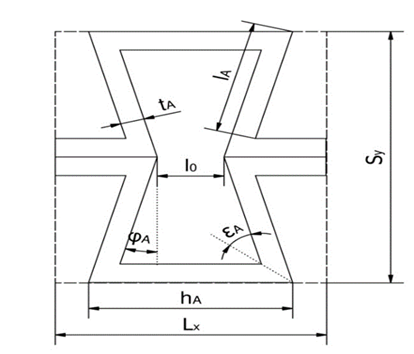Fig. 1. Elementary core cell, where: tA (mm): thickness of cell wall; lA,hA (mm): length of cell wall side, φA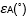: interior angles of a cell; SyLx (mm): overall dimensions of cell; l0(mm): distance between cell walls

Mathematical Models of Auxetic Cells

Analytical models of elastic constants for auxetic core cells are presented using geometry as in Fig. 1. Relative density ρA of the analyzed auxetic structure may be described as the product of core density ρA* and density of the substance forming the skeleton of the structure ρsA (Eq. 1).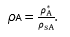(1)

Following necessary transformations relative density may be written as follows: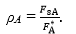(2)

The area of the elementary core section is represented by Eq. 3.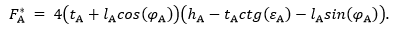(3)

The area of the substance forming the skeleton of the structure may be described as follows: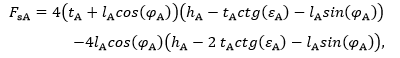(4)

Thus,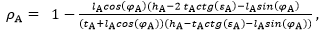(5)

where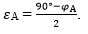(6)

The condition critical for the existence of an auxetic cell with the presented geometry is: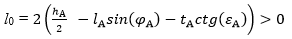(7)

and simultaneously: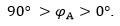(8)

Elastic constants of cells presented below are given after Masters and Evans (1996). In this paper, modeled structures and materials were characterized by linear elasticity,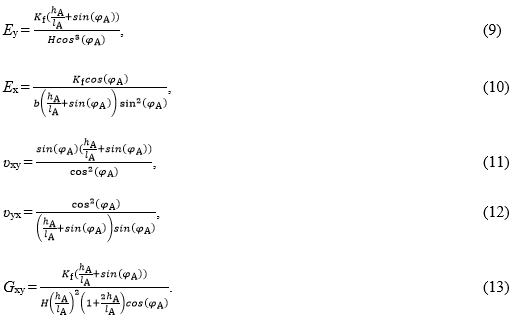where Ex (MPa) is Young’s modulus in the X axis, Ey (MPa) is Young’s modulus in the Y axis, ϑxy is Poisson’s ratio in plane XY, ϑyx is Poisson’s ratio in plane YX, Gxy (MPa) is the modulus of elasticity in shear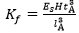(N/m) is the bending force constant, and Es (MPa) is Young’s modulus of the core substance.

Monte Carlo Optimization

In engineering computations, statistical methods constituting a component of numerical methods are used in static optimization (Smardzewski 2015). In this study, the cell shape was optimized using the Monte Carlo approach, consisting of random searches for the admissible area and on the basis of results to estimate the optimal value. In this case the maximization of the moduli of elasticity, E(MPa) and Ey (MPa), for the developed core cells was considered to be a natural optimization criterion. The set of decision variables comprised outer dimensions of cells, dimensions, and angles of cell walls. The optimal solution may be reached after a conjunctive satisfaction of numerous limiting conditions, resulting from cell shape. A significant limiting criterion was connected with the specific density of a hexagonal cell, most frequently found in honeycomb panels used in the furniture industry (= 0.0249). The mathematical model for the optimization of a polygonal auxetic cell included the following,

a) decision variables, for which the variables block Kz takes the form in Eq. 14,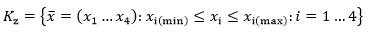(14)

where i is the number of decision variables for the cell, xtA (mm) cell wall thickness, x2 = hA (mm) length of a common cell wall, x3 = lA (mm) length of a free cell wall, and
x4 = fA (˚) is the angle of a cell wall,

b) parameter Es (MPa) Young’s modulus of the material, from which cell walls were manufactured,

c) admissible set was produced from inequality restrictions i(x) > 0i.e.: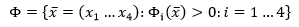(15)

The critical condition for the existence of an auxetic cell with the presented geometry was as follows: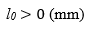(16)

The objective function as maximization of values for Young’s moduli of a cell in the primary orthotropic directions was as follows.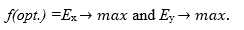(17)

The optimization program was written in the Visual Basic programming language (Microsoft, Redmond, WA, USA). A total of 30000 samplings were performed, among which approx. 0.03% were successful. Table 2 presents values of elastic constants calculated based on formulas 9 through 13 for optimized cells. In turn, Fig. 2 presents shapes of cells selected through optimization. Cells A through D are characterized by relative density identical in relation to the reference cell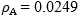. The shape of cell E made from WoodEpox® is the result of maintaining technical feasibility of the core at the maximum values of Young’s moduli and minimum relative density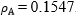. Moreover, it results from Table 2 that cell D exhibits considerable orthotropy: a high value of Young’s modulus in the direction of the Y axis, as well as a low value of Poisson’s ratio ϑyx. Greater values of elastic properties in comparison to paper cells are recorded for cell E. This is caused by a significant increase in cell wall thickness at a comparable scale of overall dimensions of the cell. Cells A through C were at comparable dimensions, Lx and Sy differ significantly in the cell wall angle. Selected cells were used in further modeling.

Table 2. Elastic Properties Provided by Mathematical Analysis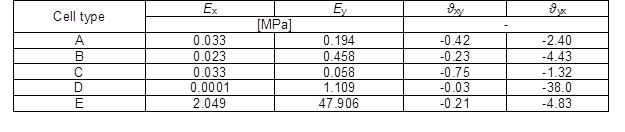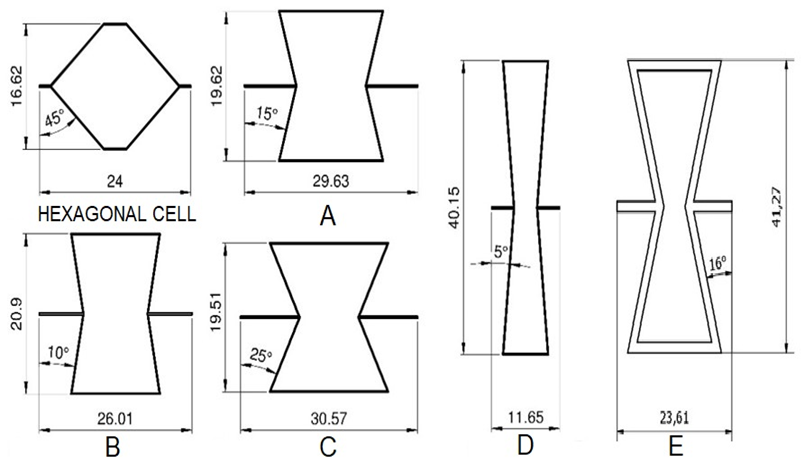Fig. 2. Elementary core cells: Hexagonal cell, the reference cell with relative density = 0.0249; A through D: paper cells; E: WoodEpox® cell

As shown, the inclination angle φdiffered within range 1 to 25°, cell length lAhA respectively within range 5 to 20 mm. The relative density of the paper cells was a fixed value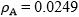, whereas relative density of the WoodEpox®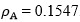.

Numerical Models

Numerical computations were performed using the Finite Element Method (FEM) applying the Abaqus v6.13 program (Dassault Systemes Simulia Corp., Waltham, MA, USA) and the resources of the EAGLE computational cluster, the Poznań Supercomputing and Networking Center, within the framework of the computational grant, “Properties of furniture panels with the synclastic surface and an auxetic core”. The computational model is presented in Fig. 3. The HEX (hexahedral elements) grid was applied along with elastic-plastic strains for selected materials (Table 1). The models were characterized by the mean number of nodes ranging from 0.6 million to 1.4 million and the mean number of elements ranging from 0.4 million to 1.2 million. The C3D6 (wedge) and C3D8R (tetrahedral) solid elements were used.

Calculations were made for loads in the direction of the X axis, and next in the Y axis for recording respective strains. For the paper models, variable loads were assumed ranging from 0 N to 0.1 N at an increment of 0.02 N for the direction of the X axis and from 0 N to 0.3 N at an increment of 0.05 N for the direction of the Y axis. For the WoodEpox ® model it was from 0 N to 10 N at an increment of 2 N and from 0 N to 35 N at an increment of 5 N. Results of calculations are given in Table 3.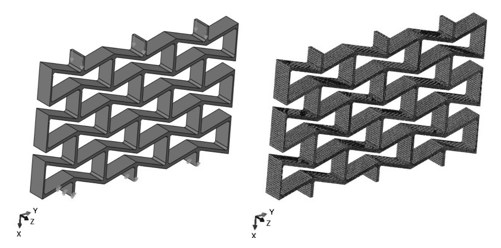Fig. 3. An example model of a core used in numerical computations; Left is a diagram of loads, and right is a mesh grid for the model

Table 3. Elastic Properties Provided by FEM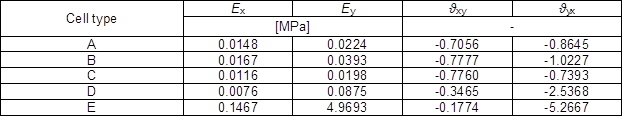As shown in Table 3, cores showed negative Poisson’s ratios. The highest Young’s moduli, E= 4.97 MPa and E= 0.15 MPa, were found for the core with cell E. It also showed the lowest value of Poisson’s ratio, ϑyx = -5.23. Among the cores with paper cells, the highest value of the modulus, Ey = 0.0875 MPa, was found for the core with cell D. At the same time, it is a model with the greatest orthotropy, since the modulus of elasticity in the direction was Ex = 0.0076 MPa. The core with cell D also showed a low value of Poisson’s ratio ϑyx = -2.54, while ϑxy = -0.35. Cores with cells A through C are characterized with evident orthotropy of elastic properties. In the case of C type cells, the values of Young’s moduli and Poisson’s ratios were very similar. This results in the core with mechanical properties close to the isotropic structure. Furthermore, a smaller angle in the wall led to a greater increase in Young’s modulus Ey(MPa), while the difference between Poisson’s ratios ϑxy and ϑyx increased as well.

Experimental Tests

In the next stage of the study, molds facilitating technical manufacture of paper cores were designed. These molds in the form of 3D solids (Fig. 4.) were designed in the Autodesk Inventor Professional® environment (Autodesk, San Rafael, CA, USA) and finished models were exported to the STL format. They were printed on a Stratasys Fortus 400mc small printer (Stratasys, Eden Prairie, MN, USA) using FDM Nylon-12™ filament (Stratasys, Minnesota, USA).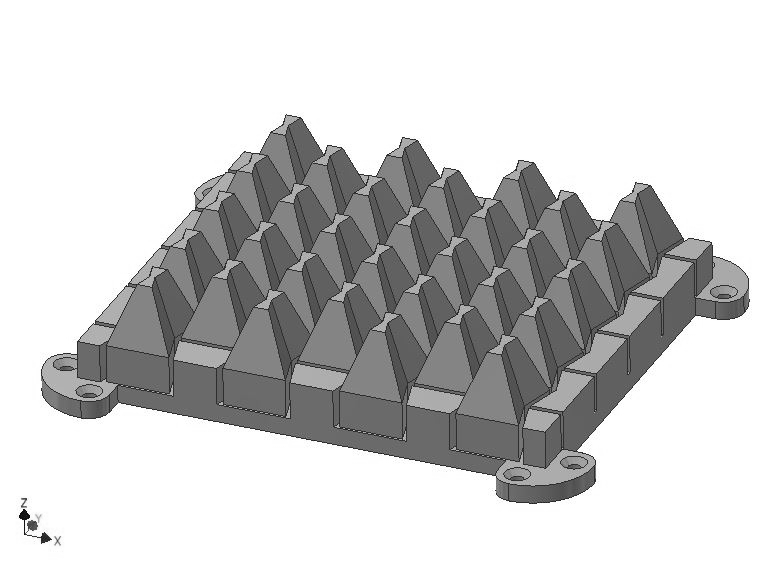Fig. 4. An example mold to manufacture the selected paper core

Sheets of paper were embossed and cut into strips of 10 mm in width using a Kongsberg-X plotter (Esko, Ghent, Belgium). The next step was to glue single strips into sets using the PVAC Woodmax FF 12.47 D2 adhesive (Synthos, Oświęcim, Poland). Such prepared sets were pulled onto the mold. The core shape was fixed using a SLW 15 laboratory dryer (Pol-Eko-Aparatura, Warsaw, Poland) at a temperature of 180 ± 1°C for 300 s. In case of the WoodEpox®, panels of 10 ± 0.2 mm in thickness were manufactured. Panels were sanded on a Felder FW 1102 perform wide belt sander (Felder Group, Żory, Poland) and milled on a Kongsberg-X milling cutter using an HM straight shank cutter of 3 mm in diameter (CMT, Poznań, Poland). Five cores each were manufactured to determine properties in planes XY and YX. Figure 5 presents the manufactured cores.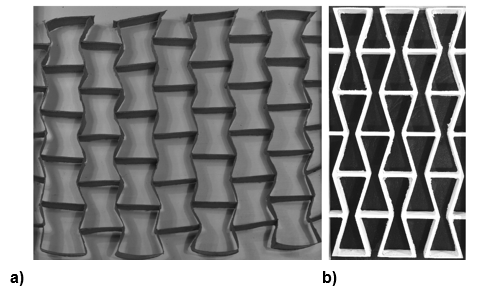Fig. 5. a) Example paper core type D, b) core E manufactured from WoodEpox®

Cores were subjected to uniaxial compression (Fig. 6). The testing stand was composed of a support beam, on which the sample and platens were placed. A ZEMIC H3-C3-25kg-3B sensor (Serial No. P2Z122353) (Zemic, Hanzhong, China) was placed between the platens, recording the force exerted onto the tested element accurate to 0.001 N. The stand was lighted with two lamps of 630 lumens to ensure proper photographic recording of the test.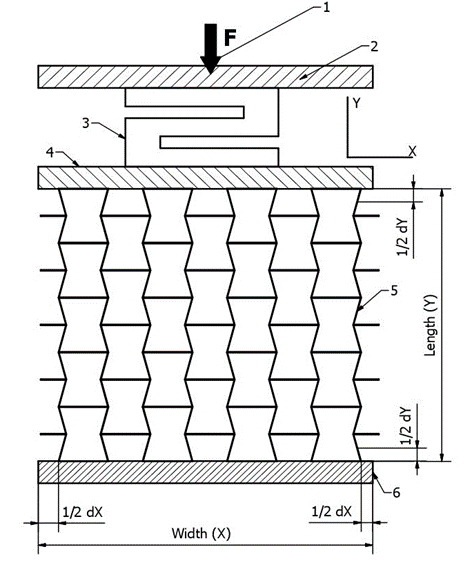Fig. 6. The testing stand: 1) pressing screw, 2) sensor platten, 3) ZEMIC H3-C3-25kg-3B sensor, 4) sample platten, 5) tested sample, and 6) support

Samples were tested following the analogical method, which was applied in numerical calculations. For each applied load a monochromatic image was taken using an Olympus OM-D camera (Olympus, Tokyo, Japan). Next strains were measured in the core by analyzing the images using the National Instruments IMAQ Vision Builder 6.1 linear analysis software (National Instruments, Austin, TX, USA) (Fig. 7).

The edge detection method was applied in digital image analysis, and respective Poisson’s ratios and Young’s moduli were calculated from the dependence shown below,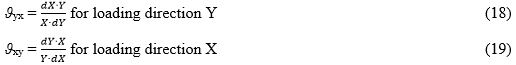where dX (mm) and dY (mm) are displacements in directions X and Y, X (mm) is width, Y (mm) is length of the core,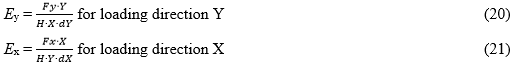where Fx,y (N) are loads for directions X and Y, H (mm) is height of the core, dX (mm) and dY (mm) are displacements in directions X and Y, respectively.